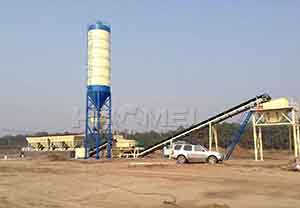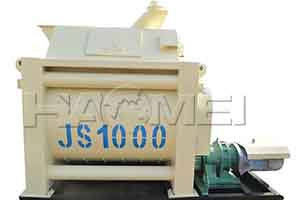No.14 Outer Ring Road, CBD, Zhengzhou, China
+86-15978414719

# How to Choose A Right Concrete Mixer for A Concrete Plant

Jan 09, 2020

If we plan to build a concrete plant with annul output of 0.35 million m3. How to choose a right concrete mixer for your concrete plant? Take Haomei HZS60 concrete batch plant for example.1. The formula to calculate annual capacity of the stationary batching plant: Qy=k*306*Qd. Here our annul output is 0.35 million, K=0.8, so the Qd is 1429.73 m3.

2. The formula to calculate daily production capacity of the stationary batching plant: Qd=0.8*C*8*Qh. Here C=3. Since the Qd is 1429.73 m3，the capacity per hour of the concrete mixing plant is 60m3/h.

3. The formula to calculate hourly production capacity of the concrete plant:

Qh=n*Q. Here n=1, Q refers to the capacity of the concrete mixer. After calculating, the Q is 60 m3/h.

4. The hourly production capacity of the mixer can be calculated according to the formula:Q=3600V1Φ/(t1+t2+t3). Here Q is hourly production capacity of mixer, V1 is feed capacity, t1 is loading time, t2 is the mixing time, t3 is concrete unloading time, Φ is discharge coefficient. The discharge coefficient is usually 0.65-0.7.

Since Q is 60 m3/h, we can know V1 is 1.9 m3,that is 1900 L. And because Φ=V2/V1. V2 is the discharging volume and it is 1300L. You need a JS1300 concrete mixer.

All these calculation is based on the standardized conditions. In the practical operation, some concrete plant needs a larger one and some needs a small one because of high efficiency. For Haomei HZS60 concrete batch plant, you just need a JS1000 concrete mixer. A smaller concrete mixer means less cost when you buy and use it.

CONTACT
1
Want to get quotation of concrete batching plant at once? Contact Us. Quick reply is as efficient as our equipment.
+86-15978414719